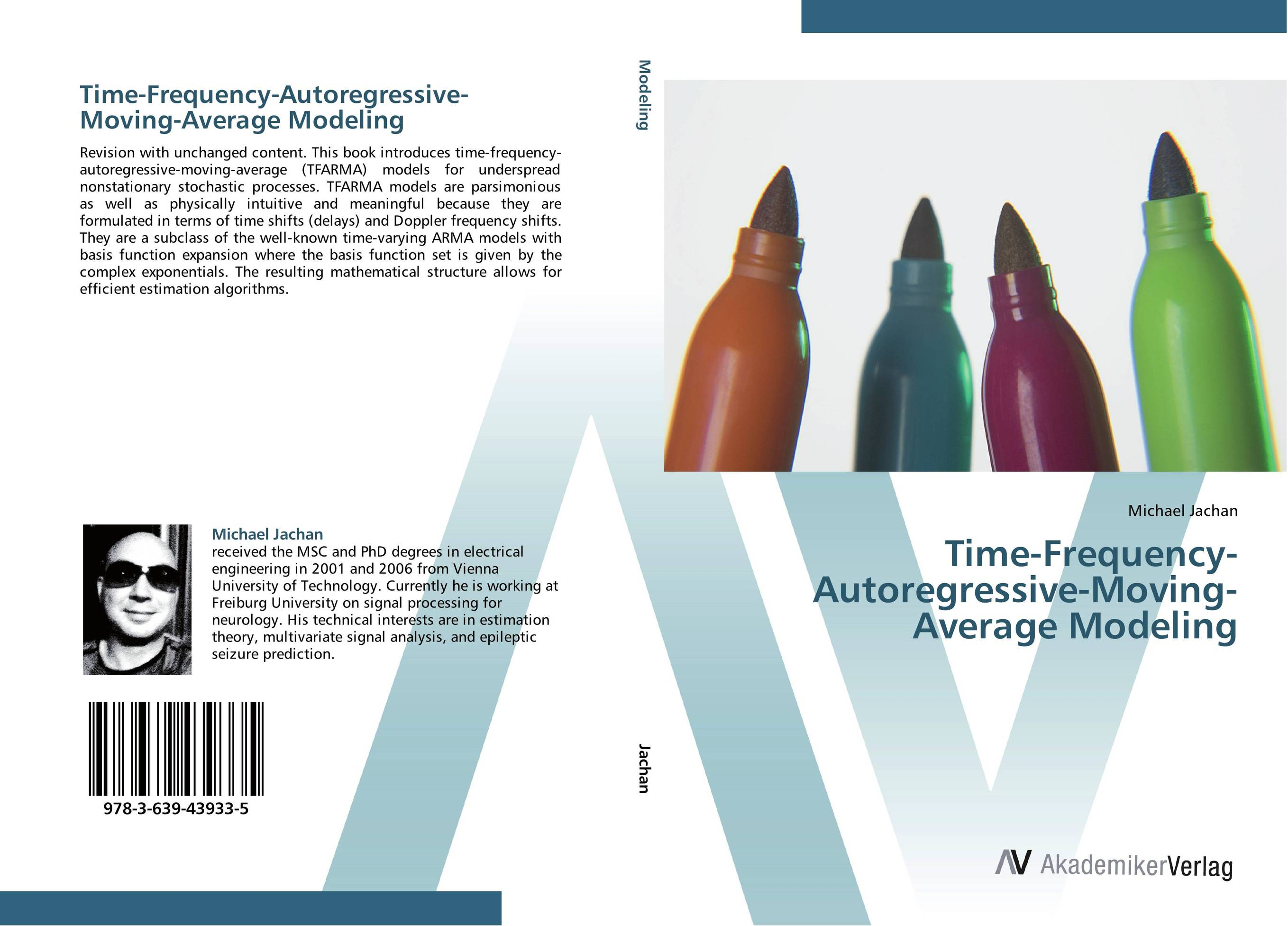# Time-Frequency-Autoregressive-Moving-Average Modeling

###### Аннотация:
Revision with unchanged content. This book introduces time-frequency-autoregressive-moving-average (TFARMA) models for underspread nonstationary stochastic processes. TFARMA models are parsimonious as well as physically intuitive and meaningful because they are formulated in terms of time shifts (delays) and Doppler frequency shifts. They are a subclass of the well-known time-varying ARMA models with basis function expansion where the basis function set is given by the complex exponentials. The resulting mathematical structure allows for efficient estimation algorithms.
###### Издания произведения:9783639439335

### Рекомендуем

#### Комментарии

Пока нет ни одного комментария
Вы должны войти для того что бы оставлять комментарии